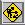# Peter Cameron's homepage

Welcome to my new homepage on GitHub.This page is under construction (and probably always will be!)

I am a quarter-time Professor in the School of Mathematics and Statistics at the University of St Andrews, and an Emeritus Professor of Mathematics at Queen Mary, University of London. In addition, I am an associate researcher at CEMAT, University of Lisbon, Portugal.

I am a Fellow of the Royal Society of Edinburgh.

### On this site### Research snapshots

#### Bases

A base for a permutation group is a sequence (a1,…,ar) of points in the permutation domain whose pointwise stabiliser is the identity. A base is irredundant if no point in the base is fixed by the stabiliser of its predecessors; it is minimal if no point in the base is fixed by the stabiliser of all the others.

A couple of things are clear:

• any minimal base is irredundant (but the converse is false);
• any re-ordering of a minimal base is minimal (but this is not true for irredundant bases).

In 1995, Dima Fon-Der-Flaass and I showed that the three conditions on irredundant bases, namely

• they are preserved by re-ordering;
• they all have the same size;
• they are the bases of a matroid;
are all equivalent. We call groups having this property IBIS groups.

Recently several people (Murray Whyte, Scott Harper, Pablo Spiga, maybe others) asked whether the set of sizes of irredundant bases form an interval. This is true. However, the analogue for minimal bases is false. For any integer d > 1, there is a group whose minimal bases have sizes 1 and d only. However, it is not yet known whether they do form an interval if the permutation group is required to be transitive.

A further question is: What happens for greedy bases? One of these is found by choosing the next base point in an orbit of maximum size for the stabiliser of its predecessors. (There is some variability because there might be a number of orbits of the same size.)

Old research snapshots are kept here.

 I am Honorary Editor-In-Chief of the Australasian Journal of Combinatorics, an international open-access journal published by the Combinatorial Mathematics Society of Australasia.School of Mathematics and Statistics University of St Andrews North Haugh St Andrews, Fife KY16 9SS SCOTLAND Tel.: +44 (0)1334 463769 Fax: +44 (0)1334 46 3748 Email: pjc20(at)st-arthurs(dot)ac(dot)uk   [oops – wrong saint!] Page revised 13 April 2023

### A problem

A talk by Peter Keevash in the Pure Maths colloquium reported his successes on formerly untouchable problems in combinatorial design theory, including the existence of t-(n,k,λ) designs (collections of k-subsets of an n set such that any t-subset lies in exactly λ of them) for all values of the parameters such that the necessary divisibility conditions are satisfied and n is sufficiently large compared with the other parameters. Also, a similar result if "set", "subset" and "cardinality" are replaced by "vector space", "subspace" and "dimension" (over a fixed finite field). And even better, an estimate for how many such designs exist. A modification of the method deals with resolvable designs.

This led me to wonder what the big remaining challenges are. Keevash's method tends to work if the blocks are of bounded size while the number of points is large. So probably the major problem now in combinatorial design theory is:

1. Which numbers n are the orders of finite projective planes?

It is widely conjectured that the answer is "prime powers, and these only", though a few years ago John Thompson proposed an alternative conjecture, that the answer is "orders of characteristically simple groups (that is, powers of orders of simple groups), and these only. In this case the number of points is about n2, while the block size is about n.

The smallest value which would distinguish between the two conjectures is of course 60, and I do not expect to see this resolved any time soon!

One can ask about the ultimate generalisation of resolvability. I will restrict myself to Steiner systems here (the case λ=1), though the problem is easily generalised.

2. Given t1<…<tm<k, suppose that the divisibility conditions for S(ti,k,n) are satisfied for 1≤im, and that n is sufficiently large. Does there exist a partition of the k-sets into S(tm,k,n) systems, each partitionable into S(tm−1,k,n) systems, and so on all the way down?

Finally, here is an ABC problem, named after the proposers, not the parameters in the problem. This comes from the synchronization project; the authors are Mohammed Aljohani, John Bamberg and I.

3. Suppose that I is a nonempty proper subset of {0,1,…,k−1}, and that A and B are are sets of k-subsets of an n-set, with sizes of pairwise intersections of sets in A belonging to I and those of its complement belonging to B. Suppose further that |A|·|B| is equal to the total number of k-sets, and that n is sufficiently large. Is it true that I or its complement must be {1,2,…,t−1)?

Note that, if this holds for I, then A is a Steiner system S(t,k,n), and B an EKR family. By Keevash's result, the Steiner system exists if the divisibility conditions hold (and n is sufficiently large.

Old problems are kept here.